# Iterating over rows and columns in Pandas DataFrame

Iteration is a general term for taking each item of something, one after another. Pandas DataFrame consists of rows and columns so, to iterate over dataframe, we have to iterate a dataframe like a dictionary. In a dictionary, we iterate over the keys of the object in the same way we have to iterate in dataframe.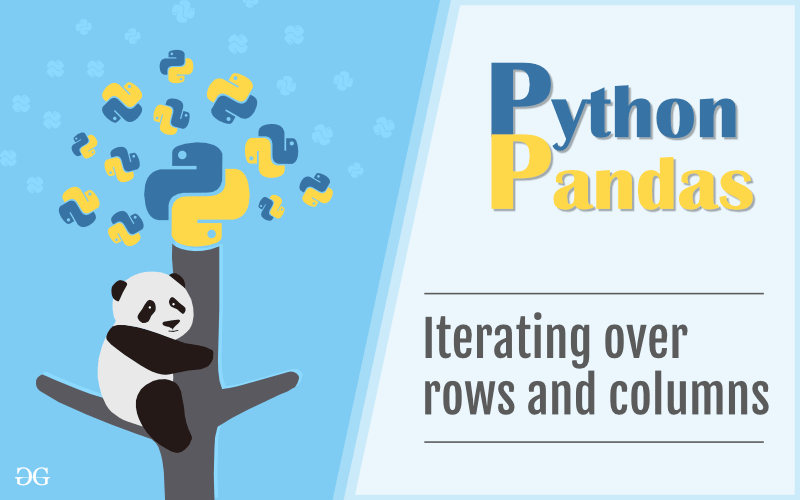## Pandas Iterate Over Rows and Columns in DataFrame

In Pandas Dataframe we can iterate an element in two ways:

• Iterating over Rows
• Iterating over Columns

## Iterate Over Rows with Pandas

In order to iterate over rows, we can use three function iteritems(), iterrows(), itertuples() . These three function will help in iteration over rows. Below are the ways by which we can iterate over rows:

• Iteration over rows using iterrows()
• Iteration over rows using iteritems()
• Iteration over rows using itertuples()

### Iteration Over Rows in Pandas using iterrows()

Example 1: Row Iteration Using iterrows()

In order to iterate over rows, we apply a iterrows() function this function returns each index value along with a series containing the data in each row.

## Python3

 `# importing pandas as pd` `import` `pandas as pd`   `# dictionary of lists` `dict` `=` `{``'name'``: [``"aparna"``, ``"pankaj"``, ``"sudhir"``, ``"Geeku"``],` `        ``'degree'``: [``"MBA"``, ``"BCA"``, ``"M.Tech"``, ``"MBA"``],` `        ``'score'``: [``90``, ``40``, ``80``, ``98``]}`   `# creating a dataframe from a dictionary` `df ``=` `pd.DataFrame(``dict``)`   `print``(df)`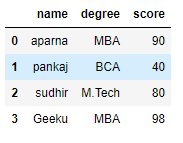Now we apply iterrows() function in order to get a each element of rows.

## Python3

 `# importing pandas as pd` `import` `pandas as pd`   `# dictionary of lists` `dict` `=` `{``'name'``: [``"aparna"``, ``"pankaj"``, ``"sudhir"``, ``"Geeku"``],` `        ``'degree'``: [``"MBA"``, ``"BCA"``, ``"M.Tech"``, ``"MBA"``],` `        ``'score'``: [``90``, ``40``, ``80``, ``98``]}`   `# creating a dataframe from a dictionary` `df ``=` `pd.DataFrame(``dict``)`   `# iterating over rows using iterrows() function` `for` `i, j ``in` `df.iterrows():` `    ``print``(i, j)` `    ``print``()`

Output: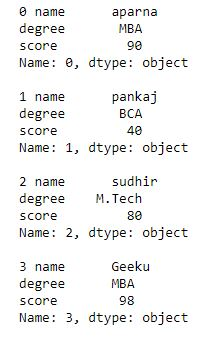Example 2: Get Each Element of Rows Using iterrows()

In this example, we are displaying the first three rows of the DataFrame using the `head(3)` method for initial data visualization.

## Python3

 `# importing pandas module` `import` `pandas as pd`   `# making data frame from csv file` `data ``=` `pd.read_csv(``"nba.csv"``)`   `# for data visualization we filter first 3 datasets` `data.head(``3``)`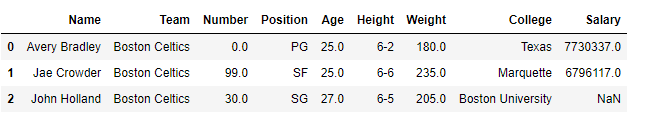Now we apply a iterrows to get each element of rows in dataframe

## Python3

 `# importing pandas module` `import` `pandas as pd`   `# making data frame from csv file` `data ``=` `pd.read_csv(``"nba.csv"``)`   `for` `i, j ``in` `data.iterrows():` `    ``print``(i, j)` `    ``print``()`

Output: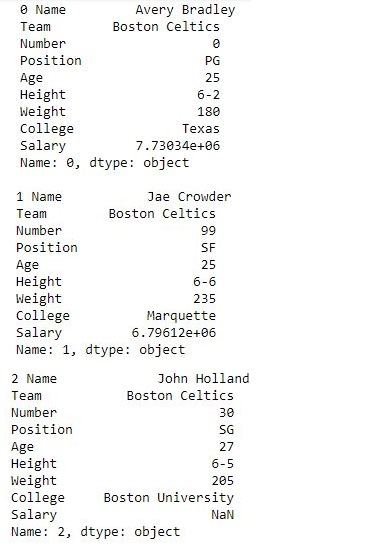### Iteration Over Rows using iteritems()

Example 1: Row Iteration Using iteritems()

In order to iterate over rows, we use iteritems() function this function iterates over each column as key, value pair with the label as key, and column value as a Series object.

## Python3

 `# importing pandas as pd` `import` `pandas as pd`   `# dictionary of lists` `dict` `=` `{``'name'``: [``"aparna"``, ``"pankaj"``, ``"sudhir"``, ``"Geeku"``],` `        ``'degree'``: [``"MBA"``, ``"BCA"``, ``"M.Tech"``, ``"MBA"``],` `        ``'score'``: [``90``, ``40``, ``80``, ``98``]}`   `# creating a dataframe from a dictionary` `df ``=` `pd.DataFrame(``dict``)`   `print``(df)`Now we apply a iteritems() function in order to retrieve an rows of dataframe.

## Python3

 `# importing pandas as pd` `import` `pandas as pd`   `# dictionary of lists` `dict` `=` `{``'name'``: [``"aparna"``, ``"pankaj"``, ``"sudhir"``, ``"Geeku"``],` `        ``'degree'``: [``"MBA"``, ``"BCA"``, ``"M.Tech"``, ``"MBA"``],` `        ``'score'``: [``90``, ``40``, ``80``, ``98``]}`   `# creating a dataframe from a dictionary` `df ``=` `pd.DataFrame(``dict``)`   `# using iteritems() function to retrieve rows` `for` `key, value ``in` `df.iteritems():` `    ``print``(key, value)` `    ``print``()`

Output: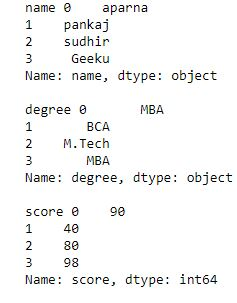Example 2: Get Each Element of Rows Using iteritems()

In this example, we are displaying the first three rows of the DataFrame using the `head(3)` method for initial data visualization.

## Python3

 `# importing pandas module` `import` `pandas as pd`   `# making data frame from csv file` `data ``=` `pd.read_csv(``"nba.csv"``)`   `# for data visualization we filter first 3 datasets` `data.head(``3``)`

Output:Now we apply a iteritems() in order to retrieve rows from a dataframe

## Python3

 `# importing pandas module` `import` `pandas as pd`   `# making data frame from csv file` `data ``=` `pd.read_csv(``"nba.csv"``)`   `for` `key, value ``in` `data.iteritems():` `    ``print``(key, value)` `    ``print``()`

Output: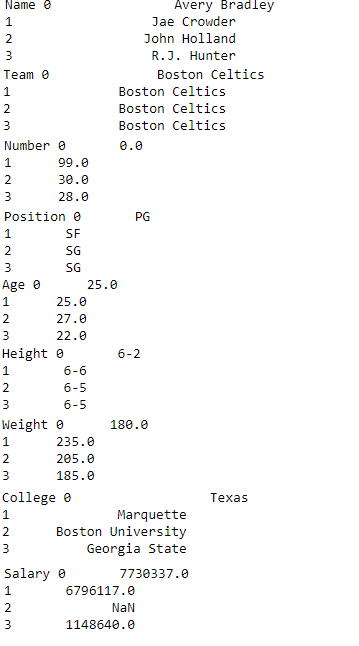### Iteration Over Rows Using itertuples()

Example 1: Row Iteration Using itertuples()

In order to iterate over rows, we apply a function itertuples() this function return a tuple for each row in the DataFrame. The first element of the tuple will be the row’s corresponding index value, while the remaining values are the row values.

## Python3

 `# importing pandas as pd` `import` `pandas as pd`   `# dictionary of lists` `dict` `=` `{``'name'``: [``"aparna"``, ``"pankaj"``, ``"sudhir"``, ``"Geeku"``],` `        ``'degree'``: [``"MBA"``, ``"BCA"``, ``"M.Tech"``, ``"MBA"``],` `        ``'score'``: [``90``, ``40``, ``80``, ``98``]}`   `# creating a dataframe from a dictionary` `df ``=` `pd.DataFrame(``dict``)`   `print``(df)`Now we apply a itertuples() function inorder to get tuple for each row

## Python3

 `# importing pandas as pd` `import` `pandas as pd`   `# dictionary of lists` `dict` `=` `{``'name'``: [``"aparna"``, ``"pankaj"``, ``"sudhir"``, ``"Geeku"``],` `        ``'degree'``: [``"MBA"``, ``"BCA"``, ``"M.Tech"``, ``"MBA"``],` `        ``'score'``: [``90``, ``40``, ``80``, ``98``]}`   `# creating a dataframe from dictionary` `df ``=` `pd.DataFrame(``dict``)`   `# using a itertuples()` `for` `i ``in` `df.itertuples():` `    ``print``(i)`

Output: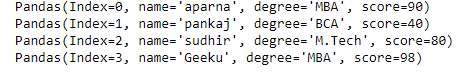Example 2: Get Each Element of Rows Using itertuples()

In this example, we are displaying the first three rows of the DataFrame using the `head(3)` method for initial data visualization.

## Python3

 `# importing pandas module` `import` `pandas as pd`   `# making data frame from csv file` `data ``=` `pd.read_csv(``"nba.csv"``)`   `# for data visualization we filter first 3 datasets` `data.head(``3``)`Now we apply an itertuples() to get atuple of each rows

## Python3

 `# importing pandas module` `import` `pandas as pd`   `# making data frame from csv file` `data ``=` `pd.read_csv(``"nba.csv"``)`   `for` `i ``in` `data.itertuples():` `    ``print``(i)`

Output: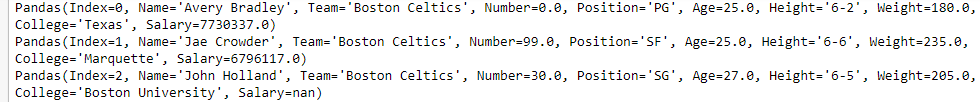## Pandas Iterate Over Columns of DataFrame

Example 1: Pandas Column Iteration

In order to iterate over columns, we need to create a list of dataframe columns and then iterating through that list to pull out the dataframe columns.

## Python3

 `# importing pandas as pd` `import` `pandas as pd`   `# dictionary of lists` `dict` `=` `{``'name'``: [``"aparna"``, ``"pankaj"``, ``"sudhir"``, ``"Geeku"``],` `        ``'degree'``: [``"MBA"``, ``"BCA"``, ``"M.Tech"``, ``"MBA"``],` `        ``'score'``: [``90``, ``40``, ``80``, ``98``]}`   `# creating a dataframe from a dictionary` `df ``=` `pd.DataFrame(``dict``)`   `print``(df)`Now we iterate through columns in order to iterate through columns we first create a list of dataframe columns and then iterate through list.

## Python3

 `# creating a list of dataframe columns` `columns ``=` `list``(df)`   `for` `i ``in` `columns:`   `    ``# printing the third element of the column` `    ``print``(df[i][``2``])`

Output: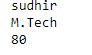Example 2: Iterating Over Rows in Pandas Python

In this example, we are displaying the first three rows of the DataFrame using the `head(3)` method for initial data visualization.

## Python3

 `# importing pandas module` `import` `pandas as pd`   `# making data frame from csv file` `data ``=` `pd.read_csv(``"nba.csv"``)`   `# for data visualization we filter first 3 datasets` `col ``=` `data.head(``3``)`   `col`Now we iterate over columns in CSV file in order to iterate over columns we create a list of dataframe columns and iterate over list

## Python3

 `# creating a list of dataframe columns` `clmn ``=` `list``(col)`   `for` `i ``in` `clmn:` `    ``# printing a third element of column` `    ``print``(col[i][``2``])`

Output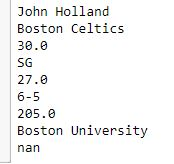Whether you're preparing for your first job interview or aiming to upskill in this ever-evolving tech landscape, GeeksforGeeks Courses are your key to success. We provide top-quality content at affordable prices, all geared towards accelerating your growth in a time-bound manner. Join the millions we've already empowered, and we're here to do the same for you. Don't miss out - check it out now!

Previous
Next